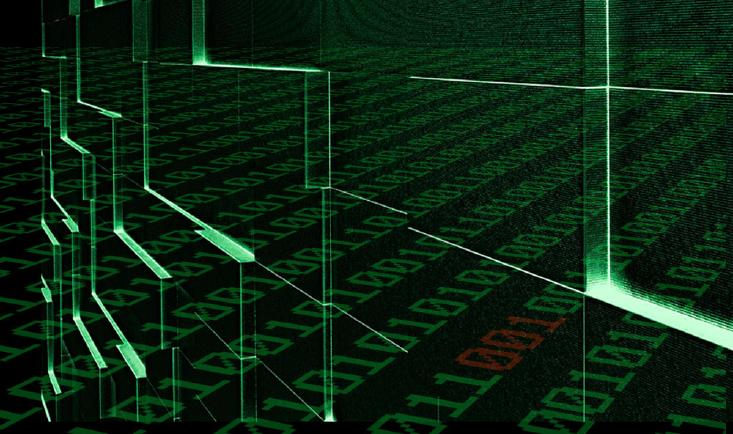• 评论

# 【NOIP试题】某科学的矩阵```#include int main() { int tot=1,n,x,y,l=0,a; //将整个二维数组置0 for(int i=0;i<100;i++){ for(int j=0;j<100;j++) a[i][j]=0; } scanf("%d",&n); //整个矩阵的中心（也就是起点）=1 a[(n-1)/2][(n-1)/2]=1; //等会操作使用的x,y指向矩阵中心 x=(n-1)/2; y=(n-1)/2; //模拟开始 while(tot<=n*n) //当计数器小于边的平方时循环 { int x1=x,y1=y; l++; //l就是本次需要加的边数 for(int i=x1;i<x1+l;i++){ x++; tot++; a[x][y]=tot; } x1=x;y1=y;//因为x,y时刻在变化，所以先设定一个变量存储本次行走的起点 for(int i=y1;i<y1+l;i++){ y++; tot++; a[x][y]=tot; } l++;//两边过后步长加大1 x1=x;y1=y; for(int i=x1;i>x1-l;i--){ x--; tot++; a[x][y]=tot; } x1=x;y1=y; for(int i=y1;i>y1-l;i--){ y--; tot++; a[x][y]=tot; } } int djx=0; //对角线之和 //打以下代码时思维比较混乱QAQ，所以代码比较乱。 for(int j=n-1;j>=0;j--){ for(int i=0;i<n;i++){ printf("%d ",a[i][j]); if(j==i) djx+=a[i][j]; //对角线判断 } printf("n");} //又写了一个模拟。。不知道当时怎么想的。。 int f=0,e=n; for(f;f<=n;f++){ e--; djx+=a[f][e]; } //因为矩阵中心元素算了两遍，所以减去一个 printf("%d",djx-a[(n-1)/2][(n-1)/2]); return 0; }```

• 近期每人完成一份基础题的解题报告
• 3,4,5月每人每月完成一份解题报告，内容以算法基础为主
• 暑假另做安排

*6月因为我们都要中考了，so……

*文章为作者独立观点，不代表少儿编程网立场

• 8小时前

• 8小时前